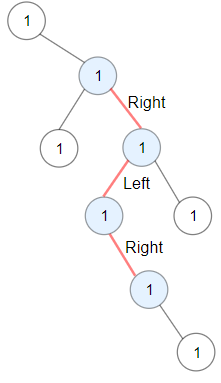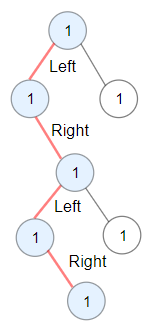Formatted question description: https://leetcode.ca/all/1372.html

# 1372. Longest ZigZag Path in a Binary Tree (Medium)

Given a binary tree root, a ZigZag path for a binary tree is defined as follow:

• Choose any node in the binary tree and a direction (right or left).
• If the current direction is right then move to the right child of the current node otherwise move to the left child.
• Change the direction from right to left or right to left.
• Repeat the second and third step until you can't move in the tree.

Zigzag length is defined as the number of nodes visited - 1. (A single node has a length of 0).

Return the longest ZigZag path contained in that tree.

Example 1:Input: root = [1,null,1,1,1,null,null,1,1,null,1,null,null,null,1,null,1]
Output: 3
Explanation: Longest ZigZag path in blue nodes (right -> left -> right).


Example 2:Input: root = [1,1,1,null,1,null,null,1,1,null,1]
Output: 4
Explanation: Longest ZigZag path in blue nodes (left -> right -> left -> right).


Example 3:

Input: root = 
Output: 0


Constraints:

• Each tree has at most 50000 nodes..
• Each node's value is between [1, 100].

Related Topics:
Dynamic Programming, Tree

## Solution 1.

// OJ: https://leetcode.com/problems/longest-zigzag-path-in-a-binary-tree

// Time: O(N)
// Space: O(H)
class Solution {
int A = 0;
pair<int, int> postorder(TreeNode* root)  {
if (!root || (!root->left && !root->right)) return { 0, 0 };
pair<int, int> ans;
if (root->left) {
auto left = postorder(root->left);
ans.first = 1 + left.second;
}
if (root->right) {
auto right = postorder(root->right);
ans.second = 1 + right.first;
}
A = max({ A, ans.first, ans.second });
return ans;
}
public:
int longestZigZag(TreeNode* root) {
postorder(root);
return A;
}
};


Java

/**
* Definition for a binary tree node.
* public class TreeNode {
*     int val;
*     TreeNode left;
*     TreeNode right;
*     TreeNode(int x) { val = x; }
* }
*/
class Solution {
public int longestZigZag(TreeNode root) {
Map<TreeNode, int[]> map = new HashMap<TreeNode, int[]>();
map.put(root, new int[]{0, 0});
int maxLength = 0;
queue.offer(root);
while (!queue.isEmpty()) {
TreeNode node = queue.poll();
int[] lengths = map.get(node);
TreeNode left = node.left, right = node.right;
if (left != null) {
int[] leftLengths = new int;
leftLengths = lengths + 1;
maxLength = Math.max(maxLength, leftLengths);
map.put(left, leftLengths);
queue.offer(left);
}
if (right != null) {
int[] rightLengths = new int;
rightLengths = lengths + 1;
maxLength = Math.max(maxLength, rightLengths);
map.put(right, rightLengths);
queue.offer(right);
}
}
return maxLength;
}
}